# Continuous Wavelet Transform (Advanced Signal Processing Toolkit)

LabVIEW 2014 Advanced Signal Processing Toolkit Help

Edition Date: June 2014

Part Number: 372656C-01

»View Product InfoDownload Help (Windows Only)

Mathematically, the continuous wavelet transform (CWT) computes the inner products of a continuous signal with a set of continuous wavelets according to the following equation: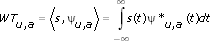where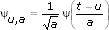WTu,a is the resulting wavelet coefficients. ψu, a denotes a continuous wavelet, where u is the shift factor and a is the scale factor of the wavelet. ψ*u,a is the complex conjugate of ψu,a. For the continuous-time signal s(t), the scale factor must be a positive real number, whereas the shift factor can be any real number. If the continuous wavelet ψu,a meets the admissibility condition, you can use the computed wavelet coefficients to reconstruct the original signal s(t).

However, you seldom use the above integration to compute the CWT because of the following reasons:

• The majority of real-world signals that you encounter are available as discrete-time samples. The analytical form of the signal s(t) usually is not accessible.
• The closed-form solution of the integration does not exist except for very special cases.

For these reasons, you usually select a set of discrete values for the scales and shifts of the continuous wavelets and then compute the CWT numerically.

Use the WA Continuous Wavelet Transform VI to compute the CWT by specifying a set of integer values or arbitrary real positive values for the scales and a set of equal-increment values for the shifts.

The following figure shows the procedure that the WA Continuous Wavelet Transform VI follows.The procedure involves the following steps:

1. Shifts a specified wavelet continuously along the time axis.
2. Computes the inner product of each shifted wavelet and the analyzed signal.
3. Dilates the wavelet based on the scale you specify.
4. Repeats steps 1 through 3 till the process reaches the maximum scale you specify.

The output of the CWT is the CWT coefficients, which reflect the similarity between the analyzed signal and the wavelets.

You also can compute the squares of the CWT coefficients and form a scalogram, which is analogous to the spectrogram in time-frequency analysis. In signal processing, scalograms are useful in pattern-matching applications and discontinuity detections. If a signal contains different scale characteristics over time, the scalogram can present a time-scale view of the signal, which is more useful than the time-frequency view of that signal. Use the WA Configure Scalogram Indicator VI to display the scalogram on an intensity graph.

The following figure shows a test signal, the Devil's Staircase fractal signal. An important characteristic of a fractal signal is self-similarity.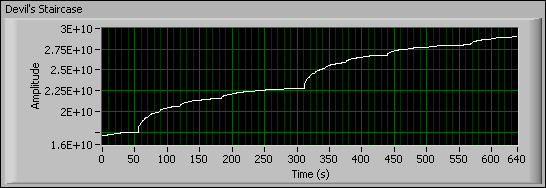The following figure shows the scalogram and the STFT spectrogram of the fractal signal, respectively.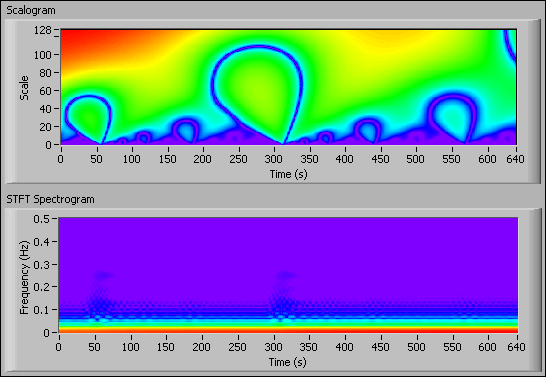In the previous figure, you can see the self-similarity characteristic of the signal clearly in the Scalogram graph but not in the STFT Spectrogram graph. The STFT Spectrogram graph displays the conventional time-frequency analysis result of the signal.

The CWT has the following general disadvantages:

• The CWT adds excess redundancy and is computationally intensive, so you usually use this transform in offline analysis applications.
• The CWT does not provide the phase information of the analyzed signal. For applications in which the phase information is useful, use the analytic wavelet transform (AWT).
• You cannot reconstruct the original signal from the CWT coefficients. For applications that require signal reconstruction, use the discrete wavelet tools.

## Application Example: Breakdown Point Detection

One useful CWT application is the detection of abrupt discontinuities or breakdown points in a signal. The following figure shows an example that detects the breakdown points in a noise-contaminated signal using the WA Continuous Wavelet Transform VI.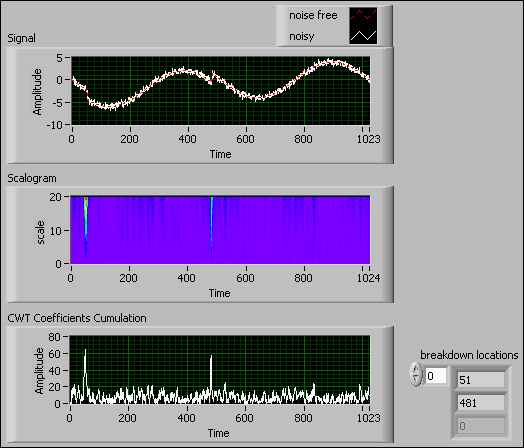The Signal graph in the previous figure shows the HeaviSine signal, which is a common wavelet test signal, contaminated with white noise. The HeaviSine signal is a sinusoid with two breakdown points—one at 51 and the other at 481. The CWT precisely shows the positions of the two breakdown points by doing the following steps:

1. Computes the CWT using the Haar wavelet.
2. Calculates the squares of the CWT coefficients of the signal and forms a scalogram, as shown in the Scalogram graph in the previous figure.
3. Cumulates the CWT coefficients along the scale axis and forms a cumulation plot, as shown in the CWT Coefficients Cumulation graph in the previous figure.
4. Detects the peak locations in the CWT Coefficients Cumulation graph. The peak locations are where the breakdown points exist.

Breakdown points and noise can generate large values in the resulting coefficients. Breakdown points generate large positive or negative coefficients at all scales. Noise generates positive coefficients at some scales and negative coefficients at other scales. If you accumulate the coefficients at all scales, the coefficients of breakdown points are enlarged while the coefficients of noise at different scales counteract one another. Therefore, the peaks in the CWT Coefficients Cumulation graph correspond only to the breakdown points.

Refer to the Breakdown Point Detection VI in the labview\examples\Wavelet Analysis\WAApplications directory for an example of breakdown point detection in a signal.